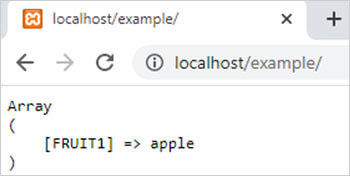PHP array_uintersect_assoc() function || PHP Array Functions# array_uintersect_assoc() function in php

1535

PHP array_uintersect_assoc() function is used to return the intersection or match values of two or more arrays by comparing the values and keys with the help of a user-defined function.

It is an inbuilt function of PHP.

Syntax:

array_uintersect_assoc(\$array1, \$array2,......, \$arrayN, user_function);

here,

• \$array1 is a PHP array which matched values returned by this function.
• \$array2,\$array3 and \$arrayN are also PHP arrays which values and keys is compared with the values  and keys of \$array1.
• user_function is a user-defined comparison function which used to calculate the common values of arrays by comparing the values and keys.

Example:

```<?php
function user_function(\$x,\$y)
{
if (\$x===\$y)
{
return 0;
}
return (\$x>\$y)?1:-1;
}
\$arr1=array("FRUIT1"=>"apple","FRUIT2"=>"guava","FRUIT3"=>"orange","FRUIT4"=>"fig");
\$arr2=array("FRUIT1"=>"apple","FRUIT2"=>"fig","FRUIT3"=>"guava","FRUIT4"=>"guava");
\$result=array_uintersect_assoc(\$arr1,\$arr2,"user_function");
echo "<pre>";
print_r(\$result);
?>```

Output:Share:## Big Hosting Days

Upto 75@ OFF on Web Hosting

## Get a .COM for just \$6.98

Secure Domain for a Mini Price